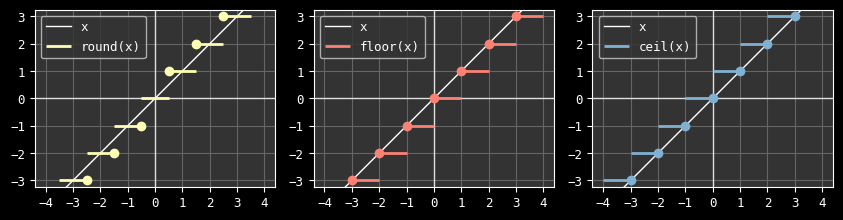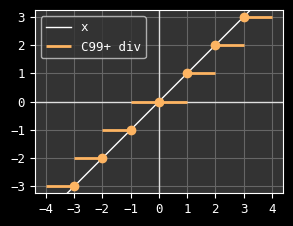# Figuring out round, floor and ceil with integer division

2022-11-25

Lately I've been transforming a float based algorithm to integers in order to make it bit-exact. Preserving the precision as best as possible was way more challenging than I initially though, which forced me to go deep down the rabbit hole. During the process I realized I had many wrong assumptions about integer divisions, and also discovered some remarkably useful mathematical properties.

This story is about a journey into figuring out equivalent functions to `round(a/b)`, `floor(a/b)` and `ceil(a/b)` with `a` and `b` integers, while staying in the integer domain (no intermediate `float` transformation allowed).

Note: for the sake of conciseness (and to make a bridge with the mathematics world), `floor(x)` and `ceil(x)` will sometimes respectively be written `⌊x⌋` and `⌈x⌉`.

## Clarifying the mission

Better than explained with words, here is how the functions we're looking for behave with a real as input:The dots indicate on which lines the stitching applies; for example `round(½)` is `1`, not `0`.

## Language specificities (important!)

Here are the corresponding prototypes, in C:

``````int div_round(int a, int b); // round(a/b)
int div_floor(int a, int b); // floor(a/b)
int div_ceil(int a, int b);  // ceil(a/b)
``````

We're going to work in C99 (or more recent), and this is actually the first warning I have here. If you're working with a different language, you must absolutely look into how its integer division works. In C, the integer division is toward zero, for both positive and negative integers, and only defined as such starting C99 (it is implementation defined before that). Be mindful about it if your codebase is in C89 or C90.

This means that in C:

``````printf("%d %d %d\n", 10/30, 15/30, 20/30);
printf("%d %d %d\n", -10/30, -15/30, -20/30);
``````

We get:

``````0 0 0
0 0 0
``````

This is typically different in Python:

``````>>> 10//30, 15//30, 20//30
(0, 0, 0)
>>> -10//30, -15//30, -20//30
(-1, -1, -1)
``````

In Python 2 and 3, the integer division is toward -∞, which means it is directly equivalent to how the `floor()` function behaves.

In C, the integer division is equivalent to `floor()` only for positive numbers, otherwise it behaves the same as `ceil()`. This is the division behavior we will assume in this article:And again, I can't stress that enough: make sure you understand how the integer division of your language works.

Similarly, you may have noticed we picked the `round` function as defined by POSIX, meaning rounding half away from `0`. Again, in Python a different method was selected:

``````>>> [round(x) for x in (0.5, 1.5, 2.5, 3.5, 4.5, 5.5, 6.5)]
[0, 2, 2, 4, 4, 6, 6]
``````

Python is following the round toward even choice rule. This is not what we are implementing here (Edit: a partial implementation is provided at the end though). There are many ways of rounding, so make sure you've clarified what method your language picked.

## Ceiling and flooring

The integer division is symmetrical around `0` but `ceil` and `floor` aren't, so we need a way get the sign in order to branch in one direction or another. If `a` and `b` have the same sign, then `a/b` is positive, otherwise it's negative. This is well expressed with a `xor` operator, so we will be using the sign of `(a^b)` (where `^` is a `xor` operator). Of course we only need to `xor` the sign bit so we could instead use `(a<0)^(b<0)` but it is a bit more complex.

Edit: note that `(a^b)` is not `> 0` when `a == b`. Also, as pointed out on lobste.rs it's likely to rely on unspecified / implementation-defined behavior (hopefully not undefined behaviour). We could use the safer `(a<0)^(b<0)` form which only generates an extra shift instruction on x86.

Looking at the graphics, we observe the following symmetries:

• `floor(x)`:
• For positive `x`, the C division works the same
• For negative `x`, the C division is one step too high (with the exception of the stitching point)
• `ceil(x)`
• For negative `x`, the C division works the same
• For positive `x`, the C division is one step too low (with the exception of the stitching point)

We can translate these observations into code using a modulo trick (which purpose is to not offset the stitching point when the division is round):

``````int div_floor(int a, int b) { return a/b - (a%b!=0 && (a^b)<0); }
int div_ceil(int a, int b)  { return a/b + (a%b!=0 && (a^b)>0); }
``````

One may wonder about the double division (`a/b` and `a%b`), but fortunately CPU architectures usually offer a division instruction that computes both at once so this is not as expensive as it would seem in the first place.

Now you also have an alternative without the modulo, but it generates less effective code (at least here on `x86-64` with a modern CPU according to my benchmarks):

``````int div_floor(int a, int b) { return (a^b)<0 && a ? (1-abs(a))/abs(b)-1 : a/b; }
int div_ceil(int a, int b)  { return (a^b)>0 && a ? (abs(a)-1)/abs(b)+1 : a/b; }
``````

Edit: note that these versions suffer from undefined behaviour in case of `abs(INT_MIN)` as pointed out by `nortti` in previous comment about `xor`.

I have no hard proof to provide for these right now, so this is left as an exercise to the reader, but some tools can be found in in Concrete Mathematics (2nd ed) by Ronald L. Graham, Donald E. Knuth and Oren Patashnik. In particular:

• the reflection properties: `⌊-x⌋ = -⌈x⌉` and `⌈-x⌉ = -⌊x⌋`
• `⌈n/m⌉ = ⌊(n-1)/m⌋+1` and `⌊n/m⌋ = ⌈(n+1)/m⌉-1`

## Rounding

The `round()` function is the most useful one when trying to approximate floats operations with integers (typically what I was looking for initially: converting an algorithm into a bit-exact one).

We are going to study the positive ones only at first, and try to define it according to the integer C division (just like we did for `floor` and `ceil`). Since we are on the positive side, the division is equivalent to a `floor()`, which simplifies a bunch of things.

I initially used a `round` function defined as `round(a,b) = (a+b/2)/b` and thought to myself "if we are improving the accuracy of the division by `b` using a `b/2` offset, why shouldn't we also improve the accuracy of `b/2` by doing `(b+1)/2` instead?" Very proud of my deep insight I went on with this, until I realized it was causing more off by ones (with a bias always in the same direction). So don't do that, it's wrong, we will instead try to find the appropriate formula.

Looking at the `round` function we can make the observation that it's pretty much the `floor()` function with the `x` offset by `½`: `round(x) = floor(x+½)`

So we have:

``````round(a/b) = ⌊a/b + ½⌋
= ⌊(2a+b)/(2b)⌋
``````

We could stop right here but this suffers from overflow limitations if translated into C. We are lucky though, because we're about to discover the most mind blowing property of integers division:This again comes from Concrete Mathematics (2nd ed), page 72.

You may not immediately realize how insane and great this is, so let me elaborate: it basically means `N` successive truncating divisions can be merged into one without loss of precision (and the other way around).

Here is a concrete example:

``````>>> n = 5647817612937
>>> d = 712
>>> n//d//d//d == n//(d*d*d)
True
``````

That's great but how does that help us? Well, we can do this now:

``````round(a/b) = ⌊a/b + ½⌋
= ⌊(2a+b)/(2b)⌋
= ⌊⌊(2a+b)/2⌋/b⌋   <--- applying the nested division property to split in 2 floor expressions
= ⌊⌊a+b/2⌋/b⌋
= ⌊(a+⌊b/2⌋)/b⌋
``````

How cute is that, we're back to the original formula I was using: `round(a,b) = (a+b/2)/b` (because again the C division is equivalent to `floor()` for positive values).

Now how about the negative version, that is when `a/b < 0`? We can make the similar observation that for a negative `x`, `round(x) = ceil(x-½)`, so we have:

``````round(a/b) = ⌈a/b - ½⌉
= ⌈(2a-b)/(2b)⌉
= ⌈⌈(2a-b)/2⌉/b⌉
= ⌈⌈a-b/2⌉/b⌉
= ⌈(a-⌈b/2⌉)/b⌉
``````

And since `a/b` is negative, the C division is equivalent to `ceil()`. So in the end we simply have:

``````int div_round(int a, int b) { return (a^b)<0 ? (a-b/2)/b : (a+b/2)/b; }
``````

This is the generic version, but of course in many cases we can (and probably should) simplify the expression appropriately.

Let's say for example we want to remap an `u16` to an `u8`: `remap(x,0,0xff,0,0xffff) = x*0xff/0xffff = x/257`. The appropriate way to round this division is simply: `(x+257/2)/257`, or just: `(x+128)/257`.

Edit: it was pointed out several times on HackerNews that this function still suffer from overflows. Though, it remains more robust than the previous version with `×2`.

## Bonus: partial round to even choice rounding

Equivalent to `lrintf`, this function provided by Antonio on Mastodon can be used:

``````static int div_lrint(int a, int b)
{
const int d = a/b;
const int m = a%b;
return m < b/2 + (b&1) ? d : m > b/2 ? d + 1 : (d + 1) & ~1;
}
``````

Warning: this only works with positive values.

## Verification

Since you should definitely not trust my math nor my understanding of computers, here is a test code to demonstrate the exactitude of the formulas:

``````#include <stdio.h>
#include <math.h>

static int div_floor(int a, int b) { return a/b - (a%b!=0 && (a^b)<0); }
static int div_ceil(int a, int b)  { return a/b + (a%b!=0 && (a^b)>0); }
static int div_round(int a, int b) { return (a^b)<0 ? (a-b/2)/b : (a+b/2)/b; }

#define N 3000

int main()
{
for (int a = -N; a <= N; a++) {
for (int b = -N; b <= N; b++) {
if (!b)
continue;

const float f = a / (float)b;

const int ef = (int)floorf(f);
const int er = (int)roundf(f);
const int ec = (int)ceilf(f);

const int of = div_floor(a, b);
const int or = div_round(a, b);
const int oc = div_ceil(a, b);

const int df = ef != of;
const int dr = er != or;
const int dc = ec != oc;

if (df || dr || dc) {
fprintf(stderr, "%d/%d=%g%s\n", a, b, f, (a ^ b) < 0 ? " (diff sign)" : "");
if (df) fprintf(stderr, "floor: %d ≠ %d\n", of, ef);
if (dr) fprintf(stderr, "round: %d ≠ %d\n", or, er);
if (dc) fprintf(stderr, "ceil: %d ≠ %d\n", oc, ec);
}
}
}
return 0;
}
``````

## Conclusion

These trivial code snippets have proven to be extremely useful to me so far, and I have the hope that it will benefit others as well. I spent an unreasonable amount of time on this issue, and given the amount of mistakes (or at the very least non optimal code) I've observed in the wild, I'm most certainly not the only one being confused about all of this.

For updates and more frequent content you can follow me on Mastodon. Feel also free to subscribe to the RSS in order to be notified of new write-ups. It is also usually possible to reach me through other means (check the footer below). Finally, discussions on some of the articles can sometimes be found on HackerNews, Lobste.rs and Reddit.